# Comparison of energy efficiency evaluation methods for fans and water pumps

Energy Efficiency Evaluation of Fans and Pump Units Research on energy-saving motors, fans, and pumps, energy-saving retrofits, and energy-saving retrofit evaluations are extremely important. The actual operating efficiency measurement of electric motors is a key technology in energy efficiency evaluation of fans and pump units. This article focuses on Fan and pump unit efficiency measurement method.

I. Current Energy Consumption Analysis of Fan Pump Industry

Fans and pumps are general-purpose mechanical power-consuming equipment with a wide range of equipment. According to statistics, the annual power consumption of fans and water pumps in China accounts for more than 30% of the total electricity consumption in the country, and accounts for about 50% of the total electricity consumption in the industry. And many equipments have low operating efficiency, outdated equipment, and great potential for energy conservation.

The rated efficiency of fans and pumps above 500kW is generally up to 80%, and the rated efficiency of supporting motors is up to 95%. Regardless of the loss of the pipe network, the rated efficiency can reach about 76%. However, relevant statistics show that the average of the fans in China The operating efficiency is only 30% to 40%. The low efficiency of the unit has many application-level issues such as improper selection, improper power configuration, unreasonable layout of the pipe network, improper adjustment, and other issues. For professional issues such as selection, improper power configuration, and unreasonable pipe network configuration, professional guidance is available. Maximum optimization. Improper adjustment, drive by frequency converter, and the use of reasonable adjustments can be greatly improved. In addition to application-level issues, driving motors, fans, pumps, etc. often run away from high-efficiency operations where load changes are large, resulting in reduced efficiency and an important factor in efficiency reduction. Researching high-efficiency equipment and ensuring high-efficiency operation within a wide range is a real problem that needs to be solved by motors, fans, and water pump plants.

Second, the traditional energy efficiency evaluation method of fan and pump unit

Fan and pump unit efficiency parameters include motor efficiency, fan efficiency, and system efficiency.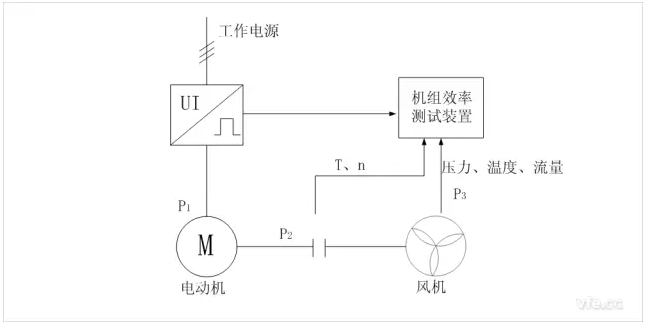Schematic diagram of traditional fan pump unit efficiency test

In the figure, the unit consists of a motor and a fan. The motor and the fan are connected coaxially. The shaft is equipped with a torque meter. The shaft power can be calculated by measuring the torque and speed. Since the loss of the coupling is negligible, it can be considered that the output power of the motor is the input power of the fan, which is represented by P2. The measurement techniques of P1 and P3 are more mature and will not be introduced here. Obviously, by obtaining P1, P2, and P3, motor efficiency, fan efficiency, and unit efficiency can be calculated. However, some fans are installed in a complete set. The torque meter cannot be installed on the shaft. The consideration in this paper is how to accurately measure P2 in this case.

Related manufacturers have developed a simple measuring device that does not require the installation of a torque meter. The device records the efficiency characteristics of various types of motors in advance, measures the input power of the motor in real time, and compares the efficiency curve to obtain the current operating efficiency of the motor. The advantage is that there is no need for a torque meter, the investment is small, and the test is convenient.

However, due to the following reasons, the accuracy is low and currently not widely used by fans and pumps:

1. There is a gap between the motor efficiency curve of the same model or the same type and the actual motor efficiency curve;

2. The efficiency characteristic curve reflects the efficiency of the rated voltage and the reference operating temperature, and the actual efficiency is greatly affected by the temperature and the voltage fluctuation of the power grid;

3, the efficiency curve provided by the motor manufacturers is the efficiency curve at rated frequency, not applicable when using frequency control.

Third, loss analysis method to measure the axial power of fans and pumps in real time

Since it is impossible to directly measure the output power of the motor. It is assumed that when the input power is known, if the loss of the motor can be known, then the input power minus the loss is the output power of the motor, and thus the operating efficiency of the motor can also be calculated. Both theory and practice have demonstrated that this idea is feasible and can achieve high accuracy. In the motor test method, this method is called loss analysis. Loss analysis is the main method for calculating the efficiency of an efficient motor.

"GB/T1032-2012 three-phase asynchronous motor test method" (hereinafter referred to as GB/T1032) pointed out: The efficiency of more than 80% of the motor should not use direct measurement of output power calculation efficiency (input-output method, referred to as A method) .

"GB18613-2012 small and medium-sized three-phase asynchronous motor energy efficiency limit value and energy efficiency rating" clear motor efficiency calculations using the measured input and output power loss analysis method (B method).

The power measurement method of the motor shaft discussed in this paper is mainly based on the loss analysis method (E1 method) of measured input power in GB/T1032. The basic idea is to use advanced test equipment, computer real-time measurement technology, database technology, use a small amount of motor factory test / type test data, connect the necessary measurement device, the fan unit as a test platform for motor energy efficiency evaluation, real-time measurement, analysis Calculate the various losses of the motor operation, and then calculate the shaft power of the motor, and on this basis, calculate the operating efficiency of the motor, fan and water pump.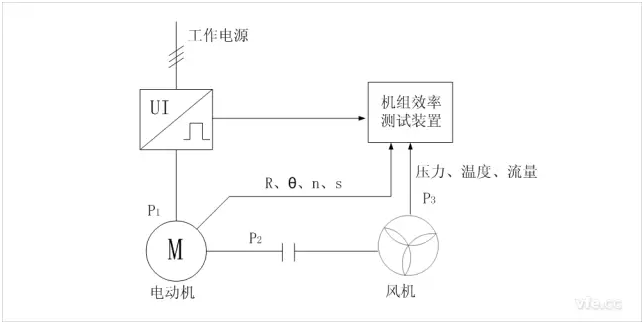Loss Analysis Method Fan Pump Unit Efficiency Tester Schematic

The loss of the motor mainly includes iron loss, wind wear, stator copper loss, copper losses and stray losses. The iron loss and the wind wear are constant losses, which are independent of the load. Stator copper losses, rotor copper losses, and stray losses are variable losses that change with load. The formula for the output power and efficiency of the motor is as follows:

Where: Î· is the motor efficiency, PFe is the iron loss, Pfw is the wind wear, Pcu1 is the stator copper loss, Pcu2 is the rotor copper loss, and Ps is the stray loss.

PFe and Pfw are obtained from the no-load test data provided when the motor is shipped.

Under no-load test at rated voltage, measured input power P0, input current I0, DC resistance R0, can obtain a constant loss Pcon;

The no-load test is performed at different input voltages. Pfe and Pfw can be separated by curve fitting technique to obtain PFe and Pfw.

In GB/T 1032, Pcu1 and Pcu2 are obtained from the load test, and the relevant data of the load test must be converted to the specified temperature calculated from the thermal test to obtain the temperature rise. The purpose of this paper is to obtain the current efficiency in real time. With the fan as the motor load, it is only necessary to calculate Pcu1 and Pcu2 under the current load. The current DC resistance of the stator must be obtained by converting the current temperature and the actual temperature of the stator winding when measuring the DC resistance.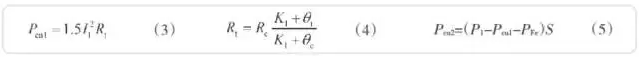I1 is the current input current, Rt is the current DC resistance of the stator winding, Rc is the measured stator winding DC resistance, and Î¸c is the stator winding temperature when measuring the DC resistance. Î˜t is the current stator winding temperature, K1 is the reciprocal of the resistance temperature coefficient of the stator winding conductor material at 0Â°C, and S is the slip rate measured in real time.

The spurious load consumption at rated power is calculated as follows: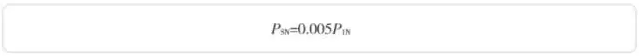Note: P1N is the input power when the rated output power, the proportion of different power motors is different. See GB/T1032 for details.

Load stray under different input power from the following formula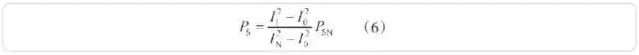I1 is the input current measured in real time, In is the rated input current, and Io is the no-load current at the rated voltage.

Fourth, the key factors affecting the accuracy of shaft power measurement

Take the rated parameter of a 10KV/500KW asynchronous motor as an example:

The motor efficiency is 95.03%, the input power is 526.12KW, and the total loss is 26.12KW. The percentage of each loss and its total loss are:

â— PFe=6.886kW (26.36%)

â— Pfw=3.949kW (15.12%)

â— Pcu1=8.533kW (32.67%)

â— Pcu2=4.12kW (4.12%)

â— Ps = 2.63kW (10.07%)

At rated power, the sum of stator copper loss, rotor copper loss, and stray loss account for approximately 60% of the total loss, and the sum of iron loss and wind wear accounts for approximately 40% of the total loss. The former is a variable loss and the latter is a constant loss. In actual operation, the motor often operates below the rated power, the constant loss does not change, and the variable loss decreases. In contrast, the constant loss accounts for a large proportion, and the constant loss cannot be measured in real time in real-time operation. (5) It can be seen that the iron loss also affects the calculation of the rotor copper loss. Therefore, the integrity and accuracy of the no-load test data are the most important factors affecting the efficiency calculation.

From equations (3)-(6), the accurate measurement of parameters such as input power, stator current, stator winding cold resistance, stator winding temperature, rotor winding temperature, and slip ratio is the key to improving the accuracy of variable loss.

Stator winding temperature or real-time DC resistance of the stator winding is an important factor in the stator copper super-computing. If the motor is embedded with a thermometer, the temperature measured by the thermometer can be used instead of the winding temperature when testing in the field. When the manufacturer tests, it is advisable to use the method of stopping the measurement of the DC resistance.

Slip rate measurement methods can be used to measure the speed and power supply frequency method, can also use the induction coil method to directly measure the slip rate. The former generally uses encoders, Hall sensors, photoelectric sensors, etc. The technology is relatively mature, the premise is the need to install auxiliary components such as encoders, gear plates, magnetic steel, reflectors, etc. on the rotating body, sometimes limited by the form of the device or the installation method. Out of service. The latter is completely free of installation, as long as the induction coil can be placed near the motor, but it is susceptible to the influence of the environmental magnetic field, and often cannot be accurately measured or even failed in the more complex electromagnetic environment.

The operating efficiency of the motor varies with changes in the load and the supply voltage. The above-mentioned parameters as well as the synchronous measurement and integral operation of the fan output power related parameters are effective methods for improving the accuracy of the efficiency. Simultaneous measurement requires that the meter measuring the above parameters output data in real time and output data-related time information. Since there are many types of parameters that the system needs to measure, it is usually a method of integration using several manufacturers' instruments, and it is difficult to meet the synchronization requirements. Considering that efficiency is the proportion of power, the average efficiency can be the proportion of energy. On the basis of synchronous measurement, the average efficiency is calculated by the ratio of the integrated value of the input and output power over a period of time, which can effectively reduce accidental errors and improve efficiency. The accuracy of the calculation.

Hunan Yinhe Electric Co., Ltd. specializes in the research of motor energy efficiency evaluation devices, and its AnyWay series motor energy efficiency evaluation products are widely used in major motor companies and third-party verification agencies throughout the country. In the course of many years in the construction of motor test benches, a wealth of measurement methods and techniques for the evaluation of motor energy efficiency have been accumulated. The TN4000 electronic torque meter developed by the company uses various advanced measurement equipment and computer measurement technology to ensure the accuracy of real-time measurement parameters. On the other hand, according to a large number of various types of motor test report data, a complete database has been established for some missing data. The prediction or correction is made to solve the real-time measurement problem of motor efficiency in the fan and the unit system. The efficiency error limit can reach 0.5% to 2%. The device can be used for the efficiency test of the fan and the unit of the fan enterprise, and it can also conveniently evaluate the energy consumption of the operating fan unit, update the user of the fan to replace the inefficient motor and high energy consumption equipment, and adopt the energy-efficient motor. And system-related power-saving equipment provides strong data support.

The unique acicular radiator,360 degree dissipation small wind resistance. High heat dissipation efficiency ensuring that the LED chip can work for 50000 hours.

Color temperature 3000-6000K

Lightâ‰¥90LM/W

Matching the 2.3.4 lane,tunnel and Landscape Lamp,floodlight dedicated lens.Ensure that intensity and uniformity.

Glare index Signification reduce the original LED Module size,removal of power supply problems.Low demands for application situation and installation personnel More suitable for the transformation of traditional lamps.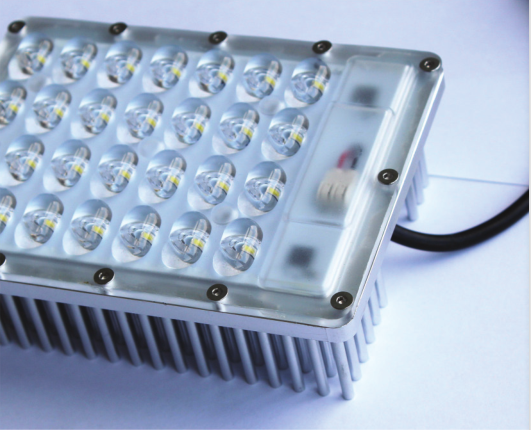Technical parameters

Power :30W,30W,15W,15W

Structure size: 135Ã—100Ã—40 mm, 208Ã—74Ã—49 mm, 125Ã—58Ã—30mm, 380Ã—40Ã—25mm

Lens size: 135Ã—100 mm, 186Ã—64 mm, 125Ã—58mm, 355Ã—40mm

Input voltage: 220 V, 220 V ,220 V, 220 V

Input current: 0.132 A, 0.132 A ,0.123 A, 0.125 A

Luminous flux: 2760 LM ,2400 LM ,1230 LM ,1300 LM

Light effect: 92 LM/W 80 LM/W 82 LM/W 86 LM/W

Color temperature: 5000 K 5000 K 5000 K 5000 K

Power factor: 0.99 PF 0.98 PF 0.98 PF 0.99 PF

Working temperature: -40+50Â°C, -40+50Â°C, -40+50Â°C ,-40+50Â°C

Led Module

Led Module,Led Modules For Signs,Led Module Bulb,Led Modules For Signage

Jiangsu chengxu Electric Group Co., Ltd , https://www.satislighting.com

Posted on